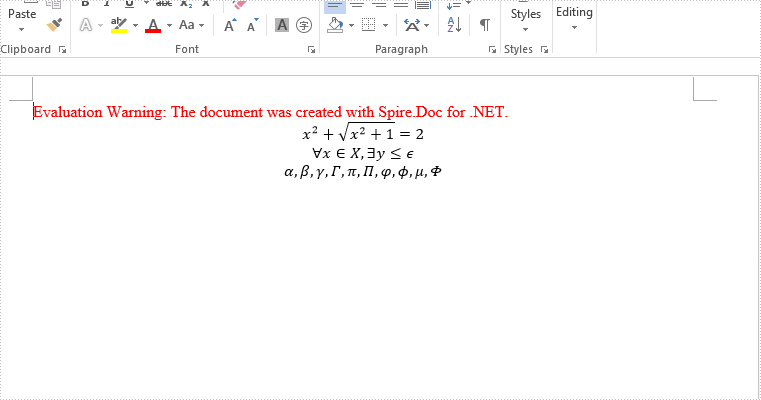Tuesday, 18 June 2019 08:21

## C# insert math equation and symbol to Word document

Written by  support iceblue
Rate this item

Starts from version 7.6.5, Spire.Doc supports to add Latex math code to Word document in C#. This article will show you how to add Latex Math Symbols and equation to word document.

//create a word document
Document doc = new Document();

//add a paragraph to the section

//add a LatexMathcode to the first paragraph
OfficeMath officeMath = new OfficeMath(doc);
officeMath.FromLatexMathCode("x^{2}+\\sqrt{x^{2}+1}=2");

//add equation to the second paragraph
OfficeMath officeMath1 = new OfficeMath(doc);
officeMath1.FromLatexMathCode("\\forall x \\in X, \\quad \\exists y \\leq \\epsilon");

//add symbols to the third paragraph
OfficeMath officeMath2 = new OfficeMath(doc);
officeMath2.FromLatexMathCode(" \\alpha,\\beta, \\gamma, \\Gamma, \\pi, \\Pi, \\phi, \\varphi, \\mu, \\Phi");

//save the document to file
doc.SaveToFile("Equation.docx", FileFormat.Docx);
System.Diagnostics.Process.Start("Equation.docx");


Effective screenshot after adding latex math code and symbols to the word document: